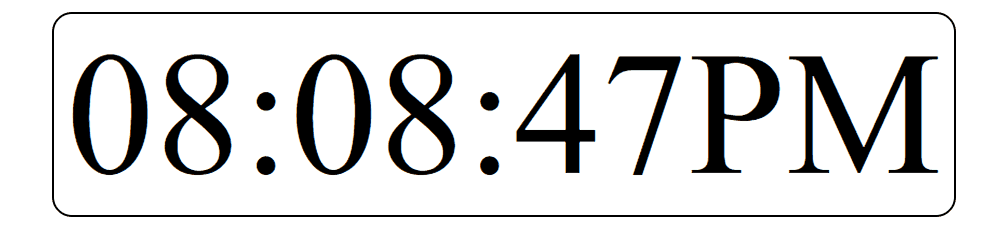# How to Design Digital Clock using JavaScript ?

Clocks are useful element for any UI if used in a proper way. Clocks can be used in sites where time is the main concern like some booking sites or some app showing arriving times of train, buses, flights, etc. Clock is basically of two types, Analog and Digital. We will be looking at making a digital one.

Approach: The approach is to use the date object to get time on every second
and then re-rendering time on the browser using the new time that we got by calling the same function each second.

HTML Code: In this section, we have a dummy time in the format of “HH:MM:SS” wrapped inside a “div” tag.

 ` ` `<``html` `lang``=``"en"``> ` `<``head``> ` `    ``<``meta` `charset``=``"UTF-8"``> ` `    ``<``meta` `name``=``"viewport"` `content``= ` `      ``"width=device-width, initial-scale=1.0"``> ` `    ``<``title``>Digital Clock ` `    ``<``link` `rel``=``"stylesheet"` `href``=``"clock2.css"``> ` ` ` `<``body``> ` `    ``<``div` `id``=``"clock"``>8:10:45 ` ` `  `    ``<``script` `src``=``"clock2.js"``> ` ` ` ` `

CSS Code: For CSS, we have just aligned our clock to the center of the page. Other than that, it is just some font-size and width which you can adjust according to your need.

 `#clock { ` `  ``font-size``: ``175px``; ` `  ``width``: ``900px``; ` `  ``margin``: ``200px``; ` `  ``text-align``: ``center``; ` `  ``border``: ``2px` `solid` `black``; ` `  ``border-radius: ``20px``; ` `} `

JavaScript Code: For JavaScript, follow the below given steps.

• Step 1: Create a function “showTime”.
• Step 2: Create an instance of the Date object.
• Step 3: Using the methods of Date object get “hours”, “minute” and “seconds”.
• Step 4: Set AM/PM depending on the hour value. The Date object works on 24-hour format so we change hour back to 1 when it get’s larger than 12. The AM/PM also changes according to that.
• Step 5: Now make a string using the same HH:MM:SS format changing the hour, minute, and second value with the values, we get from Date object methods.
• Step 6: Now replace the string variable in the “div” using innerHTML property.
• Step 7: To call the function every second use setInterval() method and set time-interval as 1000ms which is equal to 1s.
• Step 8: Now call the function at the end to start function at exact reloading/rendering time as setInterval() will call first after 1s of rendering.

Note: You can use digital fonts available online to make the clock look more beautiful. For that, you have to download their file into your project and then use the “font-face” property to use that custom font.

 `setInterval(showTime, 1000); ` `function` `showTime() { ` `    ``let time = ``new` `Date(); ` `    ``let hour = time.getHours(); ` `    ``let min = time.getMinutes(); ` `    ``let sec = time.getSeconds(); ` `    ``am_pm = ``"AM"``; ` ` `  `    ``if` `(hour > 12) { ` `        ``hour -= 12; ` `        ``am_pm = ``"PM"``; ` `    ``} ` `    ``if` `(hour == 0) { ` `        ``hr = 12; ` `        ``am_pm = ``"AM"``; ` `    ``} ` ` `  `    ``hour = hour < 10 ? ``"0"` `+ hour : hour; ` `    ``min = min < 10 ? ``"0"` `+ min : min; ` `    ``sec = sec < 10 ? ``"0"` `+ sec : sec; ` ` `  `    ``let currentTime = hour + ``":"`  `            ``+ min + ``":"` `+ sec + am_pm; ` ` `  `    ``document.getElementById(``"clock"``) ` `            ``.innerHTML = currentTime; ` `} ` `showTime(); `

Complete Code: It is the combination of the above three sections of code.

 ` ` `<``html` `lang``=``"en"``> ` ` `  `<``head``> ` `    ``<``meta` `charset``=``"UTF-8"` `/> ` `    ``<``meta` `name``=``"viewport"` `content= ` `        ``"``width``=``device``-width,  ` `        ``initial-scale``=``1``.0" /> ` ` `  `    ``<``title``>Digital Clock ` ` `  `    ``<``style``> ` `        ``#clock { ` `            ``font-size: 175px; ` `            ``width: 900px; ` `            ``margin: 200px; ` `            ``text-align: center; ` `            ``border: 2px solid black; ` `            ``border-radius: 20px; ` `        ``} ` `    `` ` ` ` ` `  `<``body``> ` `    ``<``div` `id``=``"clock"``>8:10:45 ` ` `  `    ``<``script``> ` `        ``setInterval(showTime, 1000); ` `        ``function showTime() { ` `            ``let time = new Date(); ` `            ``let hour = time.getHours(); ` `            ``let min = time.getMinutes(); ` `            ``let sec = time.getSeconds(); ` `            ``am_pm = "AM"; ` ` `  `            ``if (hour > 12) { ` `                ``hour -= 12; ` `                ``am_pm = "PM"; ` `            ``} ` `            ``if (hour == 0) { ` `                ``hr = 12; ` `                ``am_pm = "AM"; ` `            ``} ` ` `  `            ``hour = hour < ``10` `? "0" + hour : hour; ` `            ``min` `= min < 10 ? "0" + min : min; ` `            ``sec` `= sec < 10 ? "0" + sec : sec; ` ` `  `            ``let ``currentTime` `= ``hour` `+ ":"  ` `                ``+ min + ":" + sec + am_pm; ` ` `  `            ``document.getElementById("clock") ` `                ``.innerHTML` `= ``currentTime``; ` `        ``} ` ` `  `        ``showTime(); ` `    `` ` ` ` ` `  ` `

Output:My Personal Notes arrow_drop_upCheck out this Author's contributed articles.

If you like GeeksforGeeks and would like to contribute, you can also write an article using contribute.geeksforgeeks.org or mail your article to contribute@geeksforgeeks.org. See your article appearing on the GeeksforGeeks main page and help other Geeks.

Please Improve this article if you find anything incorrect by clicking on the "Improve Article" button below.

Practice Tags :

Be the First to upvote.

Please write to us at contribute@geeksforgeeks.org to report any issue with the above content.